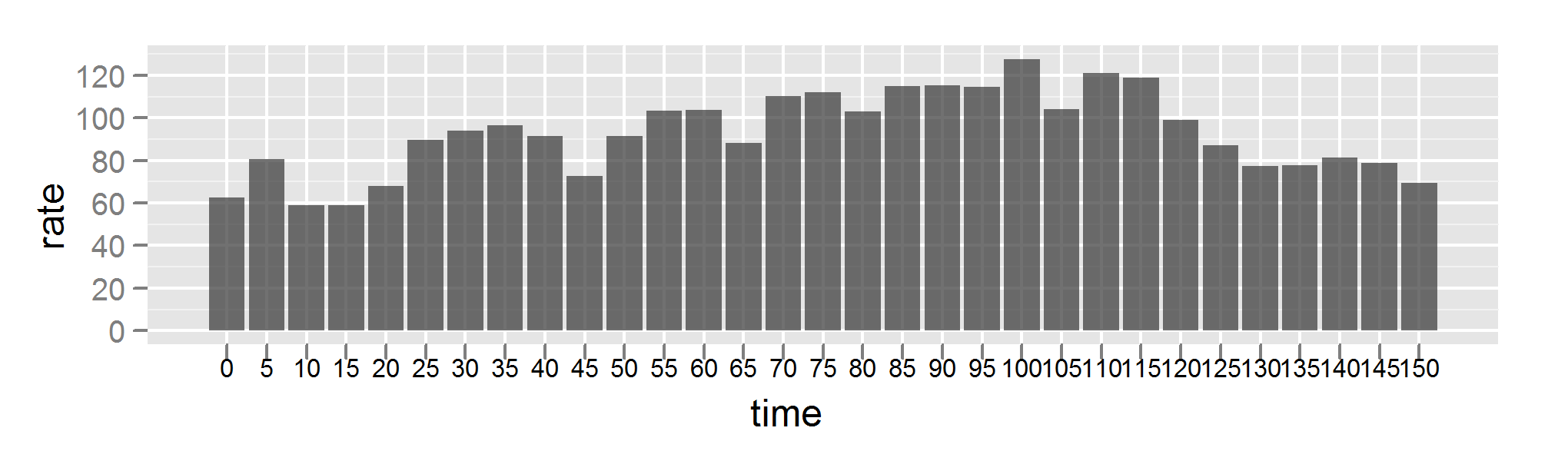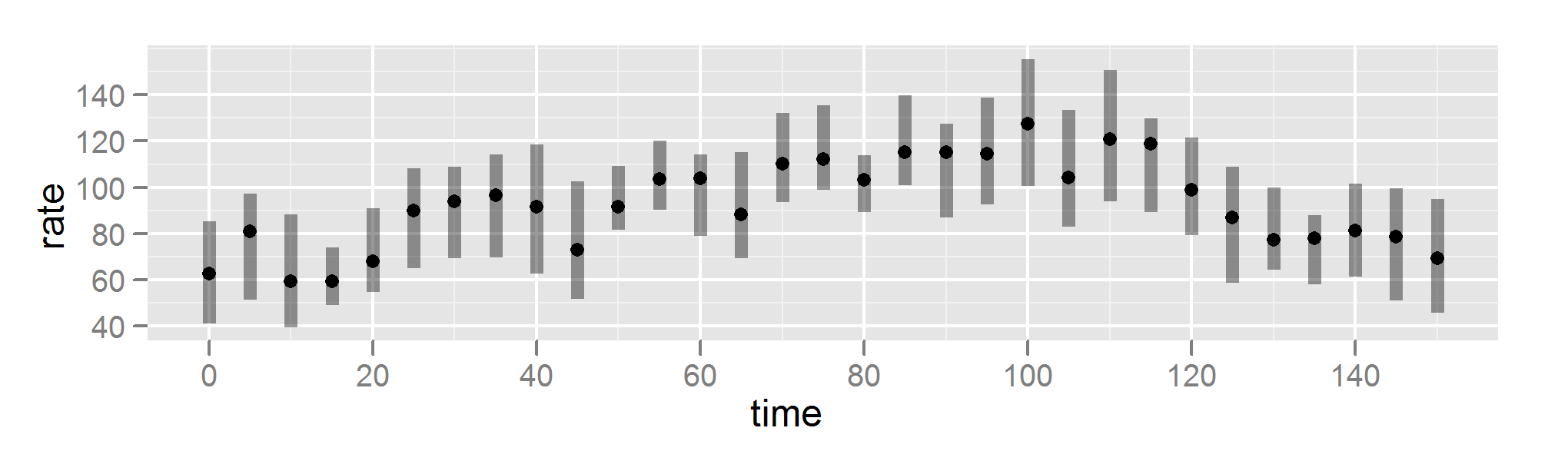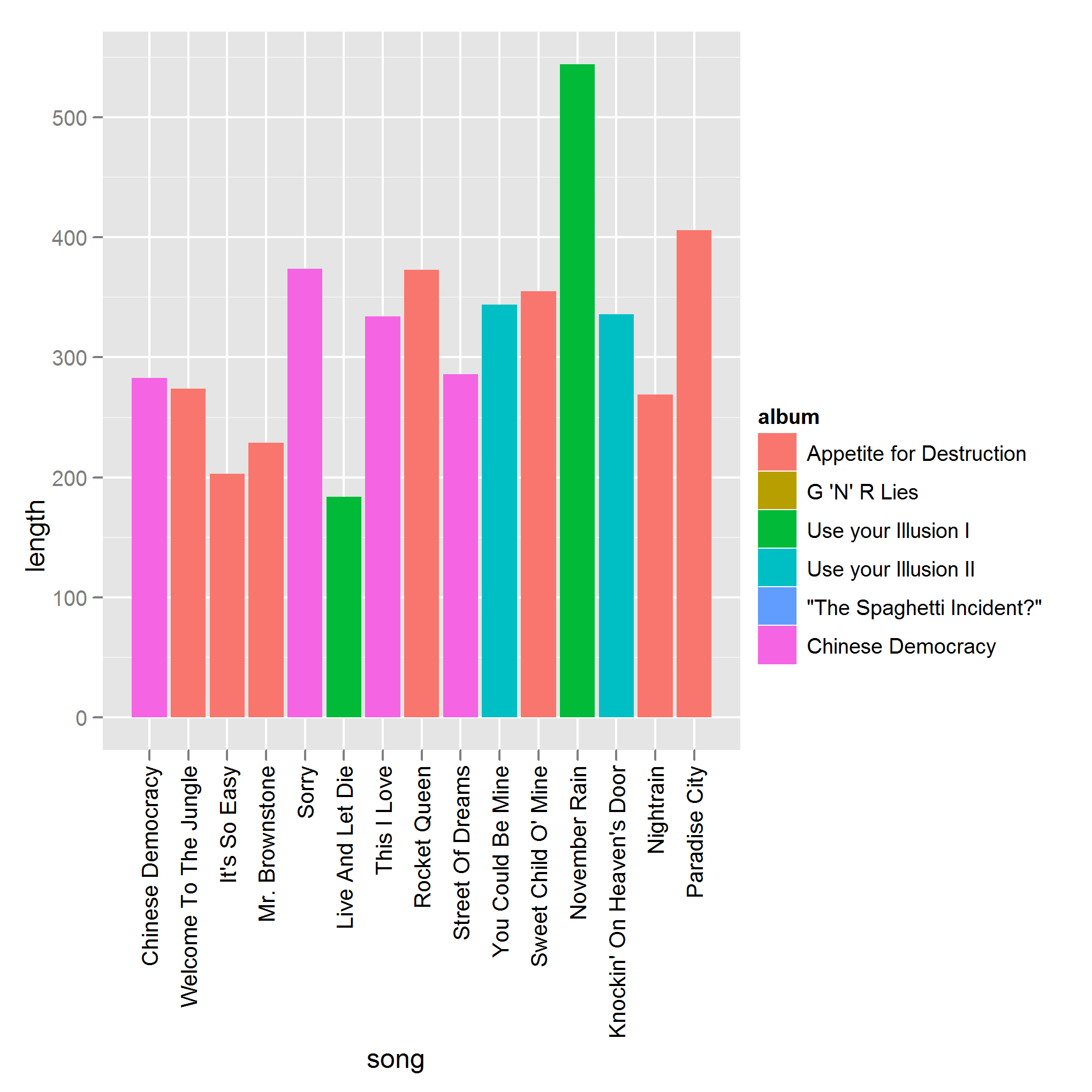Want to share your content on R-bloggers? click here if you have a blog, or here if you don't.

Last week I was asked to visualise some heart rate data from an experiment. The experimentees were clothed in protective suits and made to do a bunch of exercises while various physiological parameters were measured. Including “deep body temperature”. Gross. The heart rates were taken every five minutes over the two and a half hour period. Here’s some R code to make fake data for you to play with. The heart rates rise as the workers are made to do exercise, and fall again during the cooling down period, but it’s a fairly noisy series.
```interval <- 5 heart_data <- data.frame( +++time = seq.int(0, 150, interval) ) n_data <- nrow(heart_data) frac_n_data <- floor(.7 * n_data) heart_data\$rate = runif(n_data, 50, 80) + +++c(seq.int(0, 50, length.out = frac_n_data), +++seq.int(50, 0, length.out = n_data - frac_n_data) ) heart_data\$lower <- heart_data\$rate - runif(n_data, 10, 30) heart_data\$upper <- heart_data\$rate + runif(n_data, 10, 30)```

The standard way of displaying a time series (that is, a numeric variable that changes over time) is with a line plot. Here’s the `ggplot2` code for such a plot.

```library(ggplot2) plot_base <- ggplot(heart_data, aes(time, rate)) plot_line <- plot_base + geom_line() plot_line```Using a line isn’t always appropriate however. If you have missing data, or the data are irregular or infrequent, then it is misleading to join them together with a line. Other things are happening during the times that you have no data for. `ggplot2` will automatically removes lines that have a missing value between them (as represented by `NA` values) but in the case of irregular/infrequent data you don’t want any lines at all. In this case, using points rather than lines is the best option, effectively creating a scatterplot.

```plot_point <- plot_base + geom_point() plot_point```Since heart rate can change dramatically over the course of five minutes, the data generated by the experiment should be considered infrequent, and so I opted for the scatterplot approach.

The experimenters, however, wanted a bar chart.

```plot_bar <- plot_base + +++geom_bar(aes(factor(time), rate), alpha = 0.7) + +++opts(axis.text.x = theme_text(size = 8)) plot_bar```I hadn’t considered this use of a bar chart before, so it was interesting to think about the pros and cons relative to using points. First up, the bar chart does successfully communicate the numeric values, and the fact they they are discrete. The big difference is that the bars are forced to stretch down to zero, squeezing the data into a small range near the top of the plot. Whether or not you think this is a good thing depends upon the questions you want to answer about the heart rates.

If you want to be able to say “the maximum heart rate was twice as fast as the minimum heart rate”, then bars are great for this. Comparing lengths is what bars are made for. If on the other hand, you want to focus on the relative differences between data (“how much does the heart rate go up by when the subject did some step-ups?”), then points make more sense, since you are zoomed in to the range of the data.

There are a couple of other downsides to using a bar chart. Bars have a much lower data-ink ratio than points. Further, if we want to add a confidence region to the plot, it gets very busy with bars. Compare

```plot_point_region <- plot_point + +++geom_segment(aes( ++++++x = time, xend = time, y = lower, yend = upper), ++++++size = 2, alpha = .4) plot_point_region```

```plot_bar_region <- plot_bar + +++geom_segment(aes( ++++++x = as.numeric(factor(time)), ++++++xend = as.numeric(factor(time)), ++++++y = lower, ++++++yend = upper), size = 2, colour = "grey30") plot_bar_region```The big deal-breaker for me is that a bar chart seems semantically wrong. Bar charts are typically used to visualise a numeric variable split over several categories. This isn’t the case here: time is not categorical.

Something about this analysis was bugging me though, and I started wondering “Is it ever appropriate to use bars in a time series?”. Last night, as I was watching Guns ‘N’ Roses headline the Leeds Festival, the answer came to me. GNR were at least an order of magnitude more awesome than expected, but damn, some of those power ballads go on a long time, which allowed my mind to wander. Here’s their set list, with song lengths. (Solos and instrumentals omitted, and I wasn’t standing there with a stopwatch so data are taken from the album versions.)

```songs <- c( +++"Chinese Democracy", +++"Welcome To The Jungle", +++"It's So Easy", +++"Mr. Brownstone", +++"Sorry", +++"Live And Let Die", +++"This I Love", +++"Rocket Queen", +++"Street Of Dreams", +++"You Could Be Mine", +++"Sweet Child O' Mine", +++"November Rain", +++"Knockin' On Heaven's Door", +++"Nightrain", +++"Paradise City" )```

```albums <- c( +++"Appetite for Destruction", +++"G 'N' R Lies", +++"Use your Illusion I", +++"Use your Illusion II", +++""The Spaghetti Incident?"", +++"Chinese Democracy" )```

```gnr <- data.frame( +++song = ordered(songs, levels = songs), +++length = c(283, 274, 203, 229, 374, 184, 334, 373, 286, 344, 355, 544, 336, 269, 406), +++album = ordered(albums[c(6, 1, 1, 1, 6, 3, 6, 1, 6, 4, 1, 3, 4, 1, 1)], levels = albums) )```

```plot_gnr <- ggplot(gnr, aes(song, length, fill = album)) + geom_bar() + opts(axis.text.x = theme_text(angle = 90, hjust = 1)) plot_gnr```Here we have a “categorical time series”. The data are ordered in time, but form discrete chunks. As a bonus, the album colouring tells you which tunes have stood the test of time. In this case, the band’s debut Appetite for Destruction was played even more than the current miracle-it-arrived-at-all Chinese Democracy . G ‘N’ R Lies and “The Spaghetti Incident?”, by contrast,  didn’t feature at all.

Tagged: data-viz, r